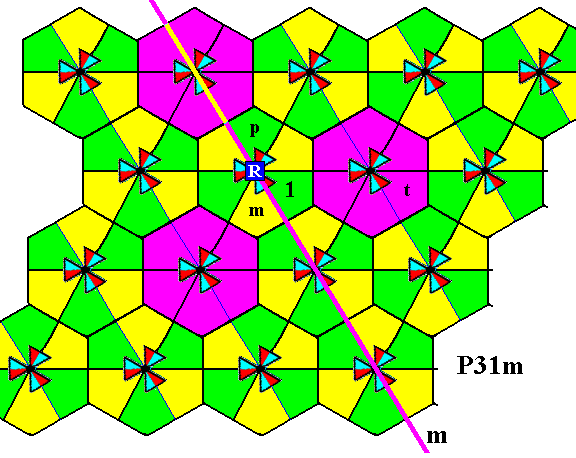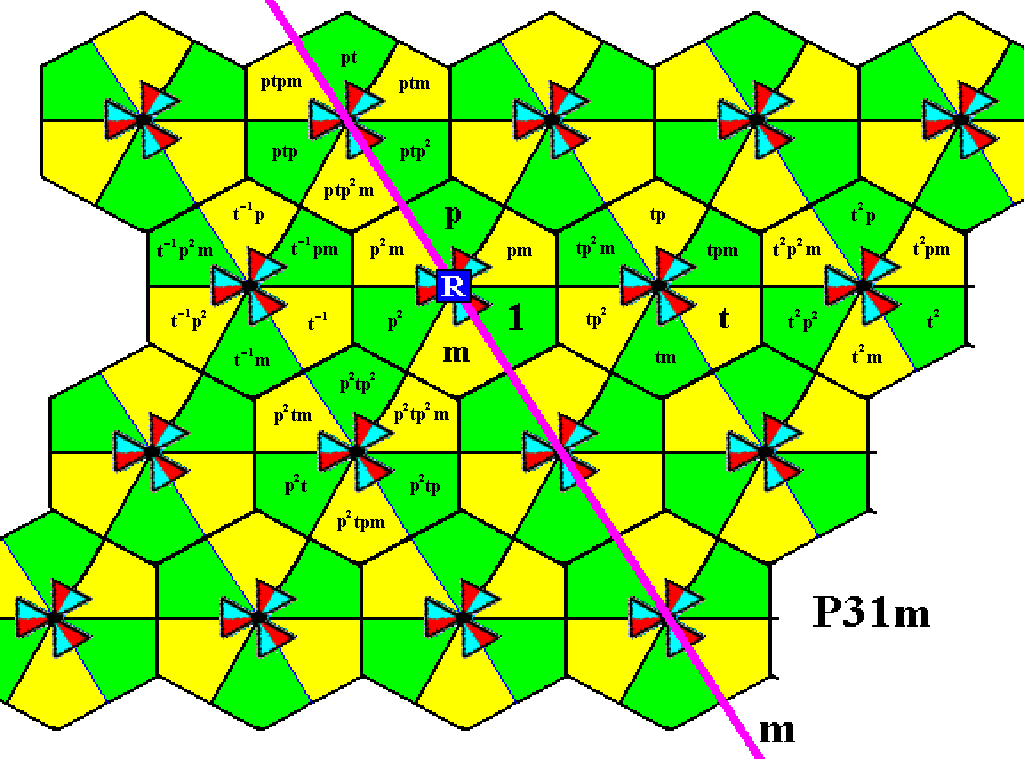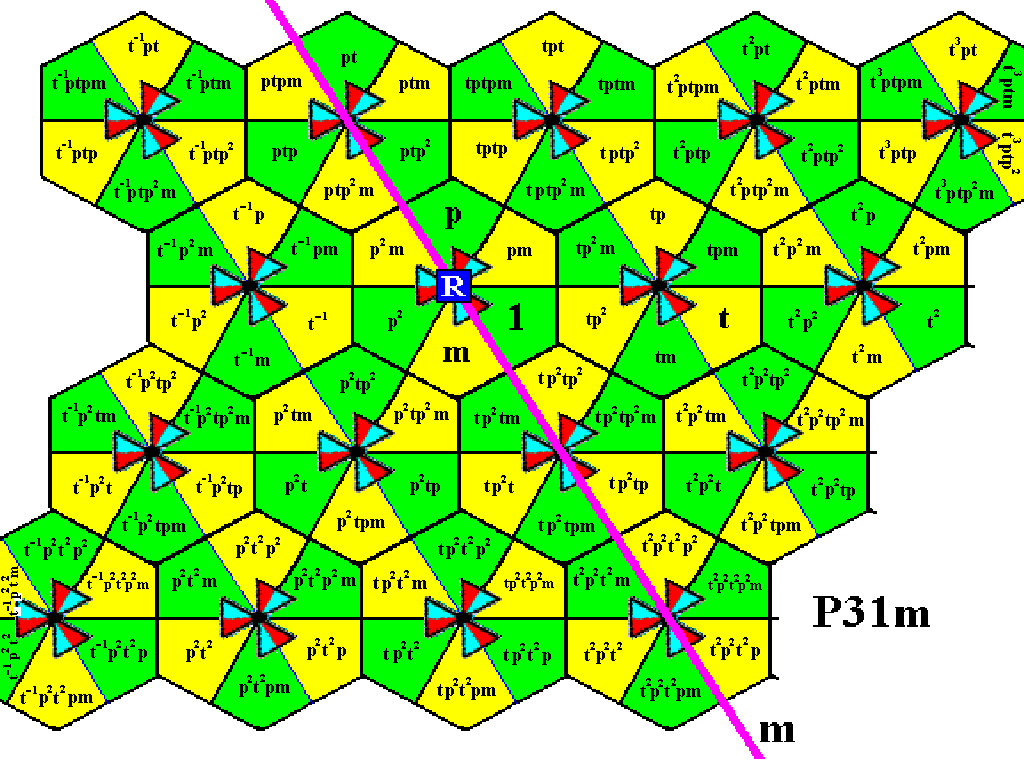```

```
Wholeness and the Implicate Order
```
```
The Crystallization Process and the Implicate Order
(Part Fifteen)

```

```
We  continue our investigation concerning the generation of group elements of the Plane Groups.
```
```
The Plane Group P31m (sequel)

In order to generate the group elements of the Plane Group P31m we will use an enlarged version of the last Figure (Figure 23) of the previous document.Figure 1.  Magnification of Figure 23 of the previous document.
The
P31m  pattern of Figure 9 of the previous document is partitioned in areas (bi-isosceles triangles) that can represent group elements (as was already done in Figure 21 of the previous document). Four such group elements are explicitly indicated :
The initial element
1  (identity element).
The generator
p ,  which is an anticlockwise rotation of 1200 about the point  R .
The generator
m ,  which is a reflection in the line  m .
The generator
t ,  which is a horizontal translation.

```
```
The next Figure shows the generation of the rest of the group elements at the lattice point  R .Figure 2.  Generation of the group elements  p2 ,  pm,   and  p2m .

```
```Figure 3.  Completion of the second row of motifs s.l., i.e. all the group elements of this row are now generated.

```
```
The next Figure indicates the rotations that can be performed on the newly generated elements, i.e. anticlockwise rotations of 1200 and 2400 about the point  R ,  in order to generate new group elements.Figure 4.  Indication of the rotations about the point  R  for generating new elements in the first and third row of motifs s.l.

```
```Figure 5.  The first and third row of motifs s.l. of our  P31m  pattern have been reached by rotations, and new group elements are generated.

```
```
These first and third rows can now be filled in by means of translations :Figure 6.  Completion of the first and third rows of motifs s.l.

```
```
The next Figure indicates how the fourth row of motifs s.l. can be reached by an anticlockwise rotation of 2400 about the point  R  -- which is the transformation  p2  -- of the elements of the last motif s.l. of the second row.Figure 7.  Indication how the elements of the fourth row of motifs s.l. can be reached by a rotation.

```
```Figure 8.  The fourth row is reached by the rotation  p2  of the elements of the last motif s.l. of the second row, about the point  R .

```
```
The fourth row can now be completed by means of translations :Figure 9.  Completion of the fourth row of motifs s.l. of the  P31m  pattern, by applying translations.

```
```
We now have generated all group elements of the displayed part of the  P31m  pattern.
```

```
In the next document we will generate the group elements of the Plane Group P6 .

e-mail :```
```
To continue click HERE for further study of the totally dynamic and holistic nature of Reality.
```
```
back to homepage

****************